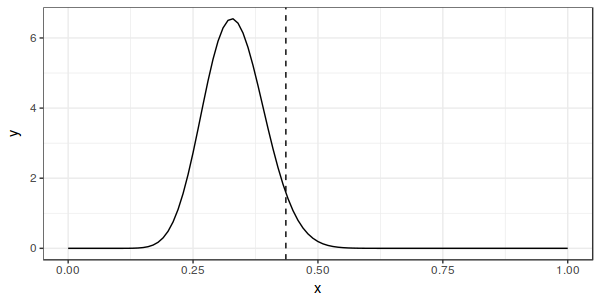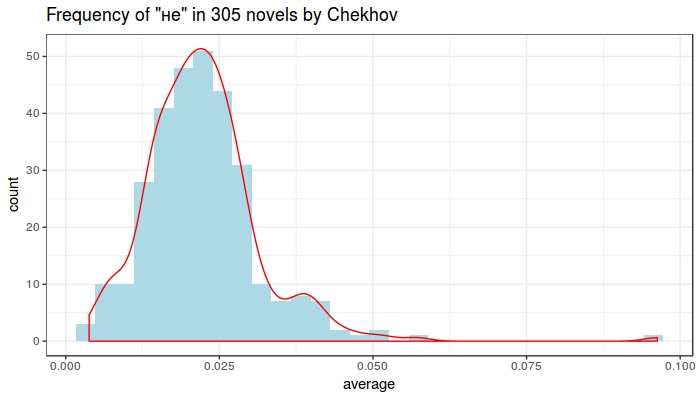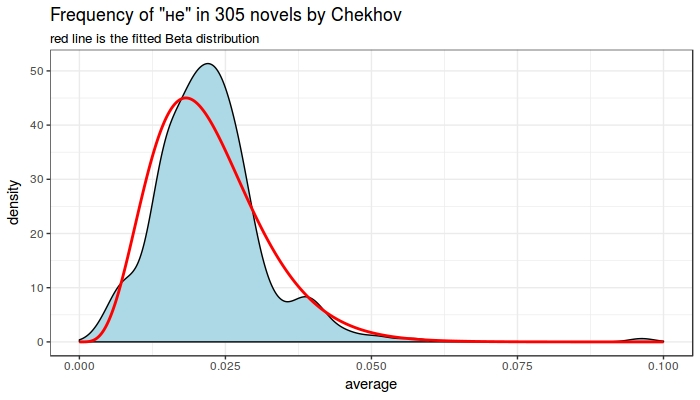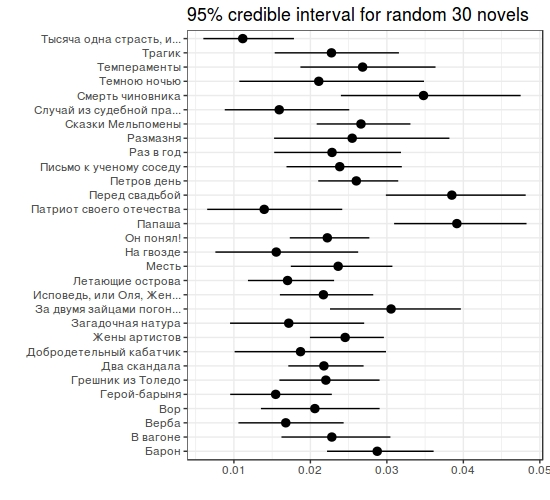Lab 14. Empirical Bayes
1. Practice with binomial distribution.
1.1 Using dbeta() and qbeta() functions visualise 95% quantile of the beta distribution with α = 20 and β = 40.1.2 Visualise two different beta distributions (α = 20, β = 40) and (α = 38, β = 43)2. Chekhov's data
I collected 311 Chekhov's novels (https://goo.gl/o18uj7)

* titles
* words
* n --- number of words in a novel
* n_words --- overall number of words in a novel

2.1 Calculate mean of the "не" words in each novel, filter out novels without "не" and create this plot.2.2 Fit the Beta destribution using mu and sigma from the destribution of "не" frequencies2.3 Calculate 95% credible interval for each novelThis content is neither created nor endorsed by Google. Report Abuse - Terms of Service